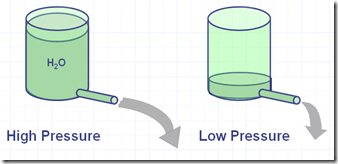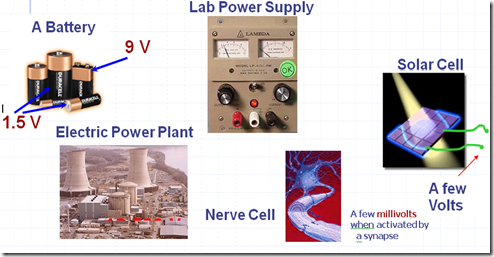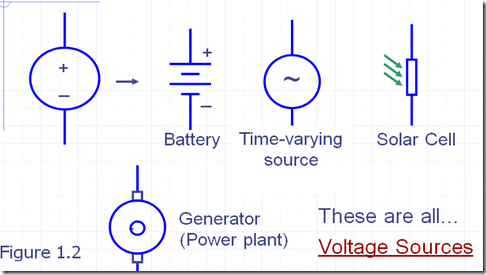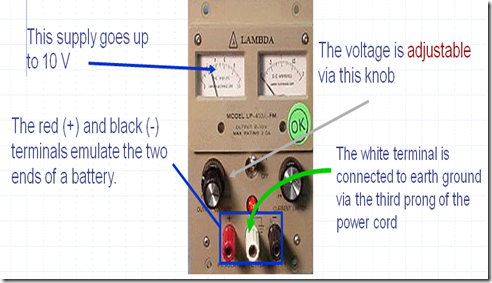### Voltage–Explanation,Production and Measurement

What is Voltage?

V = “Electrical pressure” - measured in volts.A battery in an electrical circuit plays the same role as a pump in a water system.

What Produces Voltage?

V = “Electrical pressure”Other Symbols Used for Specific Voltage SourcesA Typical Voltage Source

Lab Power SupplyRemember: A voltage is measured between two points

Measuring Voltages

We can measure voltage between

two points with a meter•Set the meter to read Voltage

• Connect the V of the meter to power supply red

• Connect COM (common) of the meter to power supply black